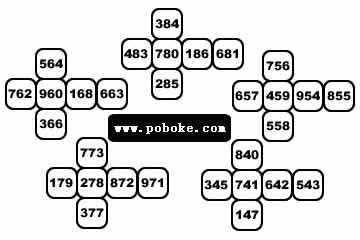## 抱歉，您的浏览器无法访问本站

### 本页面需要浏览器支持（启用）JavaScript$a_ib_ic_i(i = 1, 2, 3, 4, 5)$

$b_1 + b_2 + b_3 + b_4 + b_5 = 30 （十位数相同）$

$(a_1 + c_1) + (a_2 + c_2) + (a_3 + c_3) + (a_4 + c_4) + (a_5 + c_5) = 47 （个位与百位之和相等）$

$A = a_1 + a_2 + a_3 + a_4 + a_5$

$C = c_1 + c_2 + c_3 + c_4 + c_5$

$A = 47 - C$

$(47 - C) * 100 + 30 * 10 + C = (50 - C) * 100 + C$